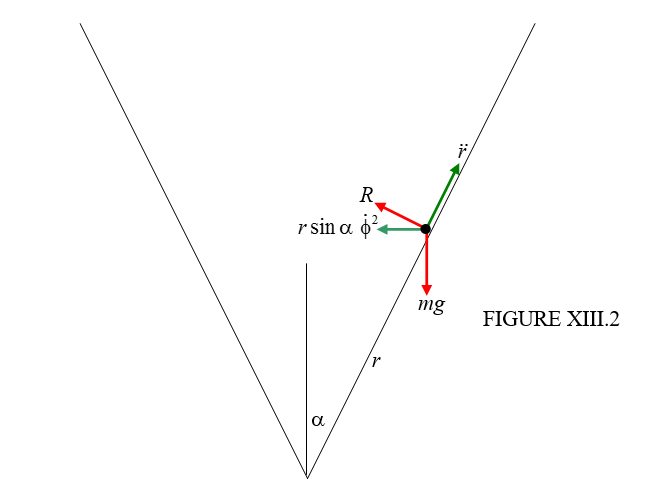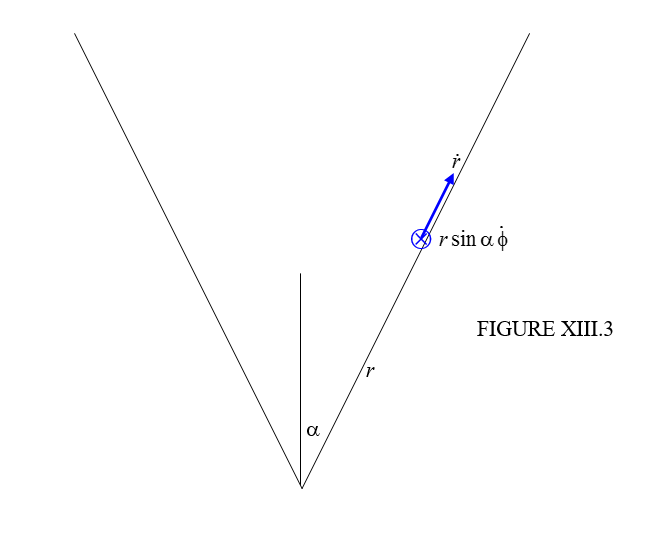$$\require{cancel}$$

# 13.6: Slithering Soap in Conical Basin

•• Contributed by Jeremy Tatum
• Emeritus Professor (Physics & Astronomy) at University of Victoria

We imagine a slippery (no friction) bar of soap slithering around in a conical basin. An isolated bar of soap in intergalactic space would require three coordinates to specify its position at any time, but, if it is subject to the holonomic constraint that it is to be in contact at all times with a conical basin, its position at any time can be specified with just two coordinates. I shall, first of all, analyse the problem with a newtonian approach, and then, for comparison, I shall analyse it using lagrangian methods. Either way, we start with a large diagram. In the newtonian approach we mark in the forces in red and the accelerations in green. See Figure XIII.2. The semi vertical angle of the cone is $$\alpha$$.The two coordinates that we need are $$r$$, the distance from the vertex, and the azimuthal angle $$\phi$$, which I’ll ask you to imagine, measured around the vertical axis from some arbitrary origin. The two forces are the weight $$mg$$ and the normal reaction $$R$$ of the basin on the soap. The accelerations are $$\ddot{r}$$ and the centripetal acceleration as the soap moves at angular speed $$\dot{\phi}$$ in a circle of radius $$r \sin \alpha$$ is $$r \sin \alpha \dot{\phi}^{2}$$.

We can write the newtonian equation of motion in various directions:

Horizontal: $$R\cos\alpha=m(r\sin\alpha\dot{\phi}^{2}-\ddot{r}\sin\alpha)$$

i.e.

$R=m\tan\alpha(r\dot{\phi}^{2}-\ddot{r}). \label{13.6.1}$

Vertical:

$R\sin\alpha-mg=m\ddot{r}\cos\alpha. \label{13.6.2}$

Perpendicular to surface:

$R-mg\sin\alpha=mr\sin\alpha\cos\alpha\dot{\phi}^{2}. \label{13.6.3}$

Parallel to surface:

$g\cos\alpha=r\sin^{2}\alpha\dot{\phi}^{2}-\ddot{r}. \label{13.6.4}$

Only two of these are independent, and we can choose to use whichever two we want to at our convenience. There are, however, three quantities that we may wish to determine, namely the two coordinates $$r$$ and $$\phi$$, and the normal reaction $$R$$. Thus we need another equation. We note that, since there are no azimuthal forces, the angular momentum per unit mass, which is $$r^{2}\sin^{2}\alpha \dot{\phi}$$, is conserved, and therefore $$r^{2}\dot{\phi}$$ is constant and equal to its initial value, which I’ll call $$l^{2}\Omega$$. That is, we start off at a distance $$l$$ from the vertex with an initial angular speed $$\Omega$$. Thus we have as our third independent equation

$r^{2}\dot{\phi}=l^{2}\Omega \label{13.6.5}$

This last equation shows that $$\dot{\phi}\rightarrow\infty$$ as $$r\rightarrow 0$$.

One possible type of motion is circular motion at constant height (put $$\ddot{r}=0$$). From Equations $$\ref{13.6.1}$$ and $$\ref{13.6.2}$$ it is easily found that the condition for this is that

$r\dot{\phi}^{2}=\frac{g}{\sin\alpha\tan\alpha}. \label{13.6.6}$

In other words, if the particle is projected initially horizontally ($$\dot{r}=0$$) at $$r=l$$ and $$\dot{\phi}=\Omega$$, it will describe a horizontal circle (for ever) if

$\Omega=(\frac{g}{l\sin\alpha\tan\alpha})^{\frac{1}{2}}=\Omega_{C}, \quad say. \label{13.6.7}$

If the initial speed is less than this, the particle will describe an elliptical orbit with a minimum $$r < l$$; if the initial speed is greater than this, the particle will describe an elliptical orbit with a maximum $$r > l$$.

Now let’s do the same problem in a lagrangian formulation. This time we draw the same diagram, but we mark in the velocity components in blue. See Figure XIII.3. We are dealing with conservative forces, so we are going to use Equation 13.4.13, the most useful form of Lagrange’s equation.We need not spend time wondering what to do next. The first and second things we always have to do are to find the kinetic energy $$T$$ and the potential energy $$V$$, in order that we can use Equation 13.4.13.

$T=\frac{1}{2}m(\dot{r}^{2}+r^{2}\sin^{2}\alpha\dot{\phi}^{2}) \label{13.6.8}$

and

$V=mgr\cos\alpha+constant. \label{13.6.9}$

Now go to Equation 13.4.13, with $$q_{i}=r$$, and work out all the derivatives, and you should get, when you apply the lagrangian equation to the coordinate $$r$$:

$\ddot{r}-r\sin^{2}\alpha\dot{\phi}^{2}=-g\cos\alpha. \label{13.6.10}$

Now do the same thing with the coordinate $$\phi$$. You see immediately that $$\frac{\partial T}{\partial\phi}$$ and $$\frac{\partial V}{\partial\phi}$$ are both zero. Therefore $$\frac{d}{dt}\frac{\partial T}{\partial\dot{\phi}}$$ is zero and therefore $$\frac{\partial T}{\partial\dot{\phi}}$$ is constant. That is, $$mr^{2}\sin^{2}\alpha\dot{\phi}$$ is constant and so $$r^{2}\dot{\phi}$$ is constant and equal to its initial value $$l^{2}\Omega$$. Thus the second lagrangian equation is

$r^{2}\dot{\phi}=l^{2}\Omega. \label{13.6.11}$

Since the lagrangian is independent of $$\phi$$, $$\phi$$ is called, in this connection, an “ignorable coordinate” – and the momentum associated with it, namely $$mr^{2}\dot{\phi}$$ is constant.

Now it is true that we arrived at both of these equations also by the newtonian method, and you may not feel we have gained much. But this is a simple, introductory example, and we shall soon appreciate the power of the lagrangian method,

Having got these two equations, whether by newtonian or lagrangian methods, let’s explore them further. For example, let’s eliminate $$\dot{\phi}$$ between them and hence get a single equation in $$r$$:

$\ddot{r}-\frac{l^{4}\Omega^{2}\sin^{2}\alpha}{r^{3}}=-g\cos\alpha. \label{13.6.12}$

We know enough by now (see Chapter 6) to write $$\ddot{r}$$ as $$v\frac{dv}{dr}$$, where $$v=\dot{r}$$ and if we let the constants $$l^{4}\Omega^{2}\sin^{2}\alpha$$ and $$g\cos\alpha$$ equal $$A$$ and $$B$$ respectively, Equation $$\ref{13.6.12}$$ becomes

$\nu\frac{d\nu}{dr}=\frac{A}{r^{3}}-B. \label{13.6.13}$

(It may just be useful to note that the dimensions of $$A$$ and $$B$$ are L4T-2 and LT-2 respectively. This will enable us to keep track of dimensional analysis as we go.)

If we start the soap moving horizontally ($$v=0$$) when $$r=l$$, this integrates, with these initial conditions, to

$\nu^{2}=A(\frac{1}{l^{2}}-\frac{1}{r^{2}})+2B(l-r). \label{13.6.14}$

Again, so that we can see what we are doing, let $$\frac{A}{l^{2}}+2Bl=C$$(note that [$$C$$] = L2T-2), and Equation $$\ref{13.6.14}$$ becomes

$\nu^{2}=C-\frac{A}{r^{2}}-2Br. \label{13.6.15}$

This gives $$\nu(=\dot{r})$$ as a function of $$r$$. The particle reaches is maximum or minimum height when $$\nu=0$$; that is where

$2Br^{3}-Cr^{2}+A=0. \label{13.6.16}$

One solution of this is obviously $$r=l$$. Of the other two solutions, one is positive (which we want) and the other is negative (which we do not want).

If we go back to the original meanings of $$A$$, $$B$$ and $$C$$, and write $$x=\frac{r}{l}$$ equation (16) becomes, after a little tidying up

$x^{3}-(\frac{l\Omega^{2}\sin\alpha\tan\alpha}{2g}+1)x^{2}+\frac{l\Omega^{2}\sin\alpha\tan\alpha}{2g}=0. \label{13.6.17}$

Recall from Equation $$\ref{13.6.7}$$ that $$\Omega_{c}=(\frac{g}{l\sin\alpha\tan\alpha})^{\frac{1}{2}}$$, and the equation becomes

$x^{3}-(\frac{\Omega^{2}}{2\Omega_{c}^{2}}+1)x^{2}+\frac{\Omega^{2}}{2\Omega_{c}^{2}}, \label{13.6.18}$

or, with $$a=\frac{\Omega^{2}}{2\Omega_{c}^{2}}$$,

$x^{3}-(a+1)x^{2}+a=0. \label{13.6.19}$

This factorizes to

$(x-1)(x^{2}-ax-a)=0. \label{13.6.20}$

The solution we are interested in is

$x=\frac{1}{2}(a+\sqrt{a(a+4)}). \label{13.6.21}$